# Perimeter and Surface Area FormulasPerimeter is the distance around a shape while surface area is the area contained within it. Daniel Grizelj / Getty Images

Perimeter and surface area formulas are common geometry calculations used in math and science. While it's a good idea to memorize these formulas, here is a list of perimeter, circumference, and surface area formulas to use as a handy reference.

### Key Takeaways: Perimeter and Area Formulas

• The perimeter is the distance around the outside of a shape. In the special case of the circle, the perimeter is also known as the circumference.
• While calculus may be needed to find the perimeter of irregular shapes, geometry is sufficient for most regular shapes. The exception is the ellipse, but its perimeter may be approximated.
• Area is a measure of the space enclosed within a shape.
• Perimeter is expressed in units of distance or length (e.g., mm, ft). Area is given in terms of square units of distance (e.g., cm2, ft2).

## Triangle Perimeter and Surface Area Formulas

A triangle is a three-sided closed figure.
The perpendicular distance from the base to the opposite highest point is called the height (h).

Perimeter = a + b + c

Area = ½bh

## Square Perimeter and Surface Area Formulas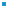Squares are four-sided figures where each side is of equal length. Todd Helmenstine

A square is a quadrangle where all four sides (s) are of equal length.

Perimeter = 4s

Area = s2

## Rectangle Perimeter and Surface Area Formulas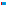A rectangle is a four-sided figure with all interior angles are right angles and opposing sides have equal lengths. Todd Helmenstine

A rectangle is a special type of quadrangle where all the interior angles are equal to 90° and all opposite sides are the same length. The perimeter (P) is the distance around the outside of the rectangle.

P = 2h + 2w

Area = h x w

## Parallelogram Perimeter and Surface Area Formulas

A parallelogram is a quadrangle where opposite sides are parallel to each other.
The perimeter (P) is the distance around the outside of the parallelogram.

P = 2a + 2b

The height (h) is the perpendicular distance from one parallel side to its opposite side.​

Area = b x h

It is important to measure the correct side in this calculation. In the figure, the height is measured from side b to the opposite side b, so the area is calculated as b x h, not a x h. If the height was measured from a to a, then the area would be a x h. Convention calls the side the height is perpendicular to the "base." In formulas, the base is usually denoted with a b.

## Trapezoid Perimeter and Surface Area Formulas

A trapezoid is another special quadrangle where only two sides are parallel to each other. The perpendicular distance between the two parallel sides is called the height (h).

Perimeter = a + b1 + b2 + c

Area = ½( b1 + b2 ) x h

## Circle Perimeter and Surface Area FormulasA circle is a path where the distance from a center point is constant. Todd Helmenstine

A circle is an ellipse where the distance from the center to the edge is constant.
Circumference (c) is the distance around the outside of the circle (its perimeter).
Diameter (d) is the distance of the line through the center of the circle from edge to edge. Radius (r) is the distance from the center of the circle to the edge.
The ratio between the circumference and the diameter is equal to the number π.​

d = 2r

c = πd = 2πr

Area = πr2

## Ellipse Perimeter and Surface Area Formulas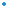An ellipse is a figure outlined by a path where the sum of the distances from two focal points are constant. Todd Helmenstine

An ellipse or oval is a figure that is traced out where the sum of the distances between two fixed points is a constant. The shortest distance between the center of an ellipse to the edge is called the semiminor axis (r1) The longest distance between the center of an ellipse to the edge is called the semimajor axis (r2).

It's actually rather difficult to calculate the perimeter of an ellipse! The exact formula requires an infinite series, so approximations are used. One common approximation, which can be used if r2 is less than three times larger than r1 (or the ellipse is not too "squished") is:

Perimeter ≈ 2π [ (a2 + b2) / 2 ]½

Area = πr1r2

## Hexagon Perimeter and Surface Area Formulas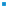A regular hexagon is a six sided polygon where each side is of equal length. Todd Helmenstine

A regular hexagon is a six-sided polygon where each side is of equal length. This length is also equal to the radius (r) of the hexagon.

Perimeter = 6r

Area = (3√3/2 )r2

## Octagon Perimeter and Surface Area Formulas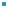A regular octagon is a eight sided polygon where each side is of equal length. Todd Helmenstine

A regular octagon is an eight-sided polygon where each side is of equal length.

Perimeter = 8a

Area = ( 2 + 2√2 )a2

Format
mla apa chicago